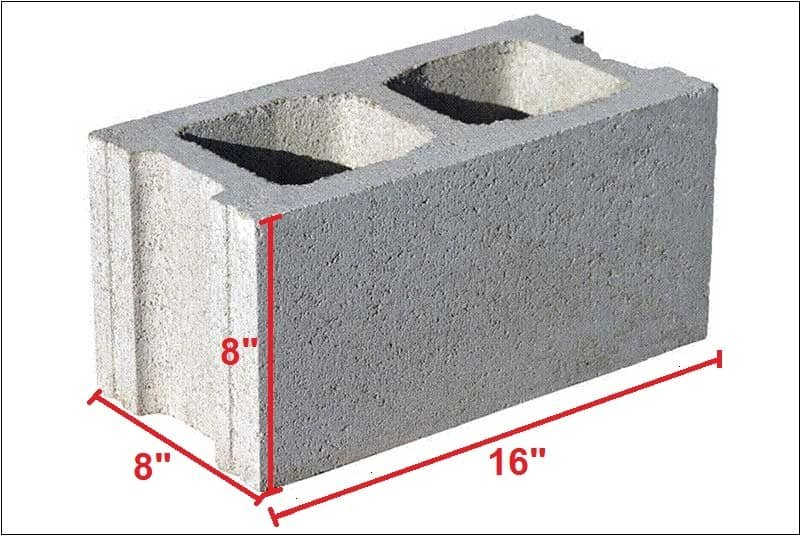# How To Calculate Number Of Concrete Blocks In A Wall

Contents

## Number Of Concrete Blocks In A Wall:

There are several methods for calculating number of blocks in a wall. In this article, I will explain two simple methods to find out total no. of blocks for a wall.

The two methods are are as follows:

1. Surface area method.
2. Volume method.

### 1. Calculation By Surface Area Method:

#### Required Data:

1. Surface area of the wall.

2. Surface area of a standard concrete block.

3. Surface area of openings in the wall.

Step 1:

Determine The Length & Height Of The Wall:

Let, the Length of the wall = 10 feet.

The height of the wall = 10 feet.

Step 2:

Calculate The Surface Area Of The Wall:

We know, Surface Area = Length x Height

∴ Surface area of the wall = 10 x 10 = 100 sq. feet.

Step 3:

Calculate The Surface Area Of Openings:

If the wall has any openings such as doors, windows, etc, calculate the surface area of openings.

Let the wall has a window of 3′ x 3′

∴ Surface area of the window = 3 x 3 =9 sq. ft

Step 4:

Subtract The Surface Area Of Openings From Surface Area Of Walls:

∴ Final Surface Area of wall = 100 – 9 = 91 sq.ft.

Step 5:

Calculate The Surface Area Of A Concrete Block With Mortar Allowance:

Let the block is 16″ x 8″ x 8″ and mortar allowance is 1″

∴ Surface Area of one block with mortar = (16+1) x (8+1)= 153 inch²

= 153/12×12 = 1.0625 sq.ftStep 6:

Divide The Total Surface Area Of The Wall By Surface Area Of One Block:

∴ Number of blocks = Surface Area of wall/Surface Area of a block = 91/1.0625 = 86

Consider 5% wastage of concrete blocks.

So the total numbers of blocks required = 86 + (86 x 5/100) = 86 + 4 = 90.

## 2. Calculation By Volume Method:

### Required Data:

1. Volume of the wall.

2. Volume of a standard concrete block.

3. Volume of openings in the wall.

Step 1:

Determine The Width, Height, And Thickness Of The Wall:

Let, the width of the wall = 10 feet.

The height of the wall = 10 feet.

The thickness of the wall = 8″ = 0.67 feet. [ Thickness of wall will be same as the thickness of one concrete block]

Step 2:

Calculate The Volume Of The Wall:

We know, Volume = Width x Height x Thickness

∴ Volume of the wall = 10 x 10 x 0.67 = 67 cubic feet.

Step 3:

Calculate The Volume Of Openings:

If the wall has any openings such as door, windows, etc, calculate the volume of openings

Let the wall has a window of 3′ x 3′ x 0.67′ [ Thickness of the window will be the same as the thickness of the wall]

∴ Volume of the window = 3 x 3 x 0.67 =6 cu. ft

Step 4:

Subtract The Volume Of Openings From Volume Of Walls:

∴ Final volume of wall = 67 – 6 = 61 cu.ft.

Step 5:

Calculate The Volume Of A Concrete Block With Mortar Allowance:

Let the block is 16″ x 8″ x 8″ and mortar allowance is 1″

∴ The volume of one block with mortar = (16+1) x (8+1) x 8 = 1224 inch3 [The mortal will be given on the upper side and one side of every concrete block]

= 1224/12x12x12 = 0.70 cu.ft

Step 6:

Divide The Total Volume Of The Wall By Volume Of One Block:

∴ Number of blocks = Volume of wall/Volume of a block = 61/0.70 = 86

Consider 5% wastage of concrete blocks.

So the total numbers of blocks required = 86 + (86 x 5/100) = 86 + 4 = 90.

Note:

If the wall does not have any opening skip step 4, and just divide the surface area of the wall by the surface area of one block.### 19 thoughts on “How To Calculate Number Of Concrete Blocks In A Wall”

1. Dear sir:
thanks

• Thank you, keep supporting

• I am pleased to learn what you are sharing to the world. This is a good knowledge

2. Nice thing to learn

3. It is really easy to calculate concrete blocks for a wall using the guideline you have shared.

Thanks for sharing.

Liton

4. Hi, I am a contractor pls let me know about estimation & calculator of METERIELS

5. Pls let me know about building meteriels & estimation

6. I enjoy ur teaching and help, guilding us to in provide more

7. Nice sir
I like it

8. how to find out the scaffolding and what is rate for this scaffolding in market ?

9. So good, if some of the request in the comment aanswered will be best.

10. Using the Surface Area Method, I don’t understand how you got the 12 in step 5

153/12×12 = 1.0625 sq.ft.

Thanks.

• 153 Ich2 was converted to square foot
in each 1 inch there’s 0.0833 feet
d(ft)/12 is the formula for converting an inch to feet
but here we have Inch2
therefore, 153/12×12 = 1.0625 Sq.ft.

11. nice Discribition

12. Thanks very much for this lectures on this topic estimation of construction material.